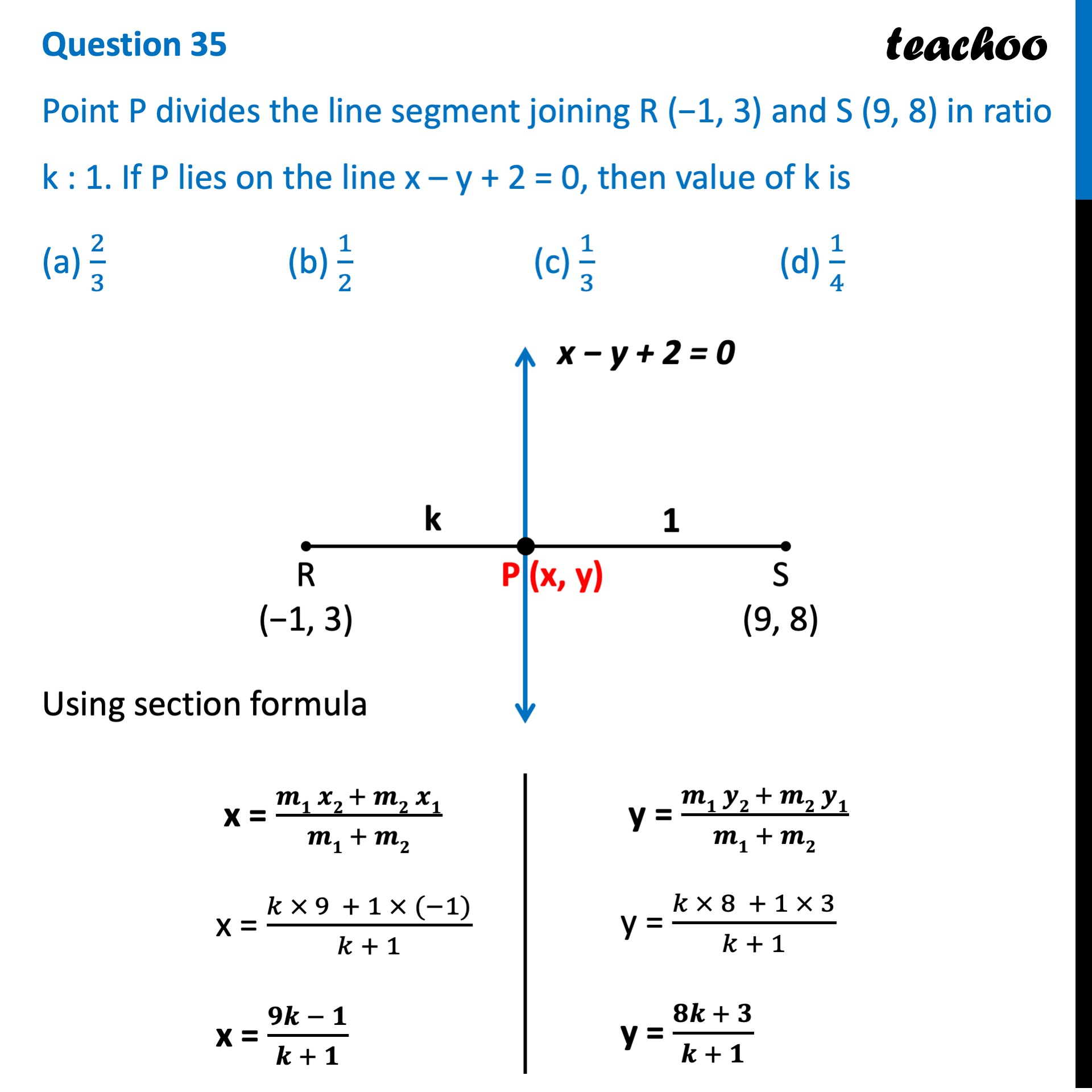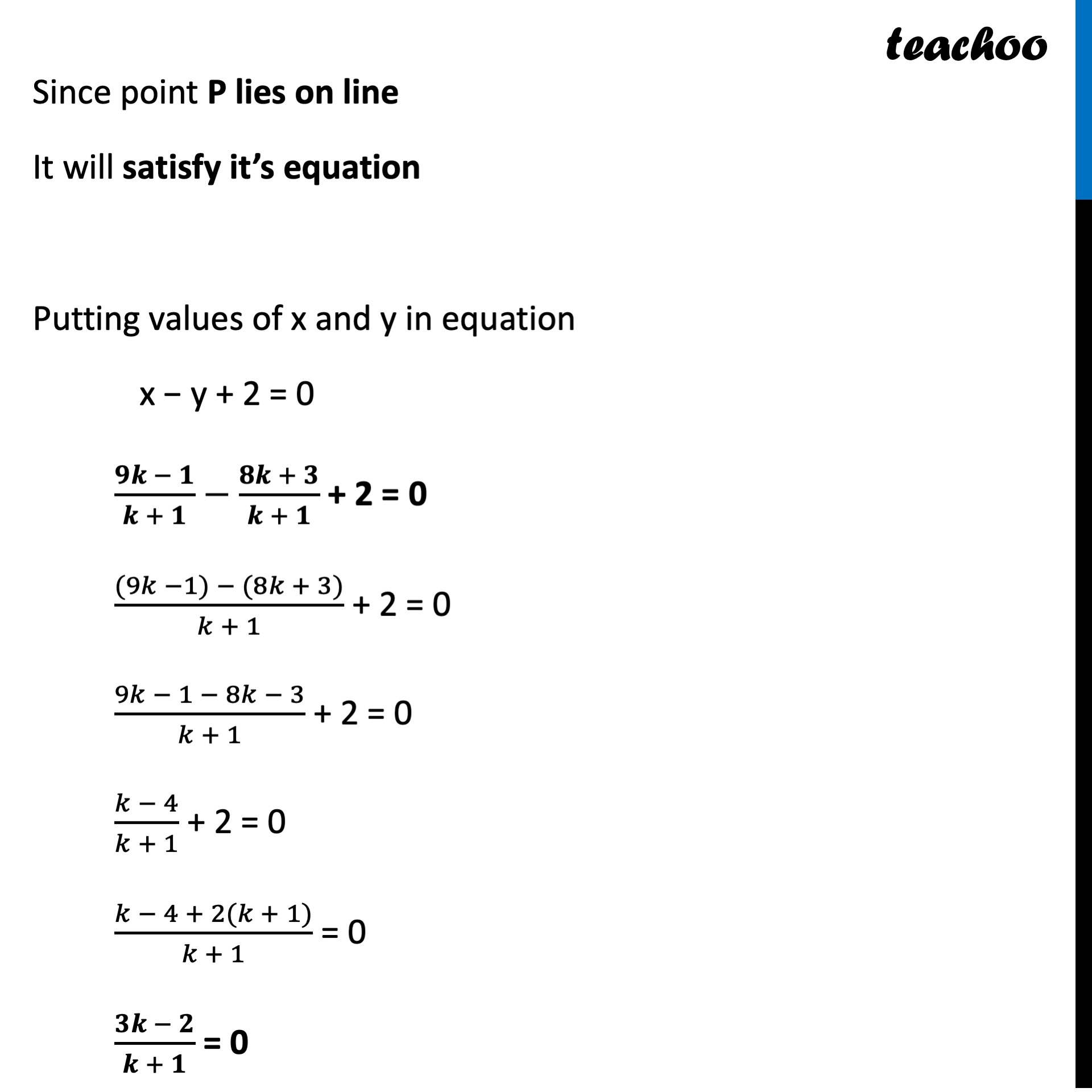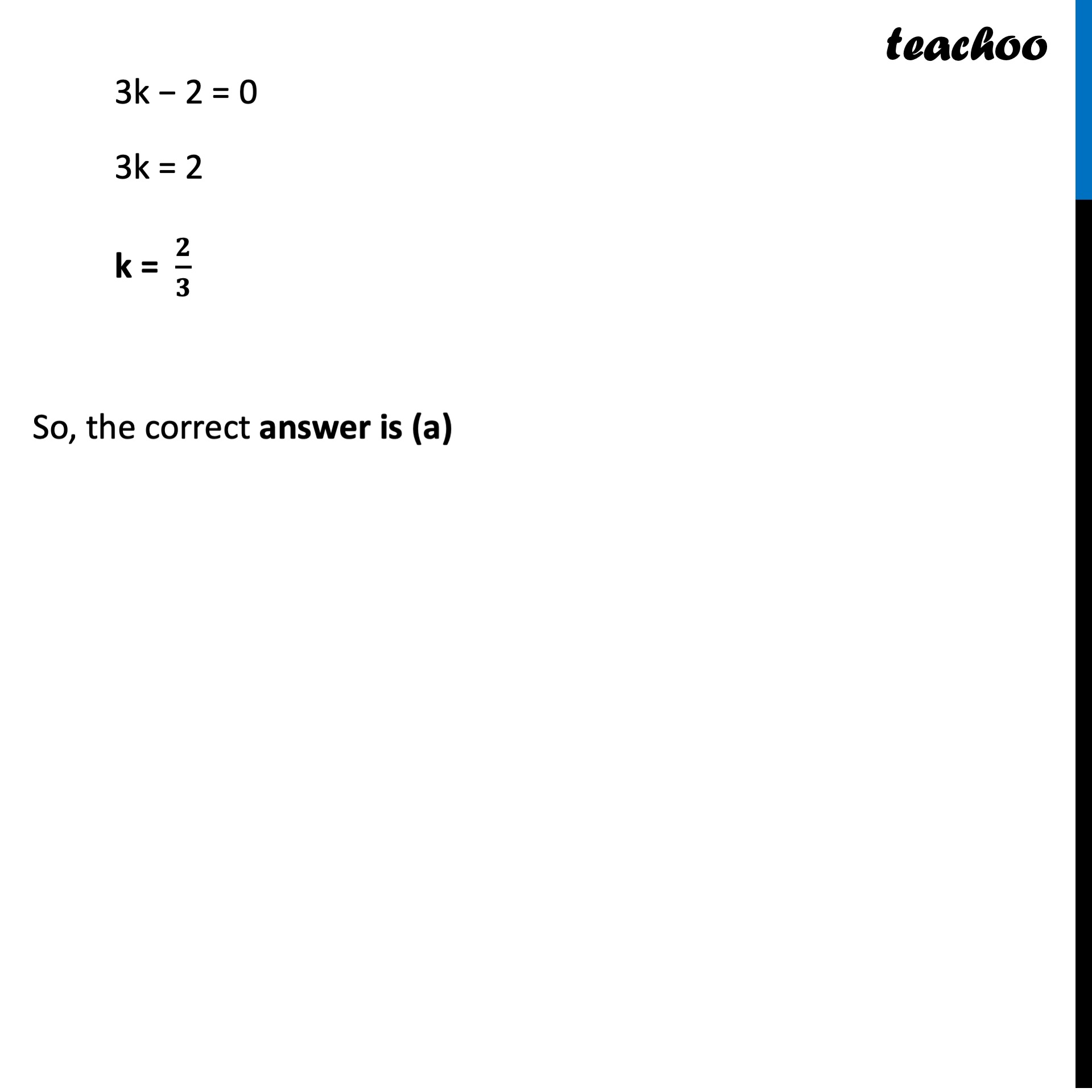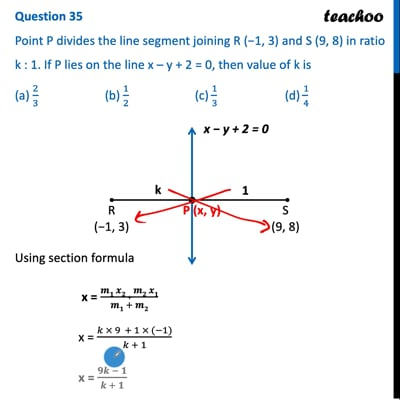CBSE Class 10 Sample Paper for 2022 Boards - Maths Standard [MCQ]

Class 10
Solutions of Sample Papers for Class 10 Boards

## Point P divides the line segment joining R (−1, 3) and S (9, 8) in ratio k:1. If P lies on the line x – y + 2 = 0, then value of k is (a) 2/3  (b) 1/2   (c) 1/3    (d) 1/4This video is only available for Teachoo black users

Introducing your new favourite teacher - Teachoo Black, at only ₹83 per month

### Transcript

Question 35 Point P divides the line segment joining R (−1, 3) and S (9, 8) in ratio k:1. If P lies on the line x – y + 2 = 0, then value of k is (a) 2/3 (b) 1/2 (c) 1/3 (d) 1/4 Using section formula x = (𝒎𝟏 𝒙𝟐 + 𝒎𝟐 𝒙𝟏)/(𝒎𝟏 + 𝒎𝟐) x = (𝑘 × 9 + 1 × (−1))/(𝑘 + 1) x = (𝟗𝒌 − 𝟏)/(𝒌 + 𝟏) y = (𝒎𝟏 𝒚𝟐 + 𝒎𝟐 𝒚𝟏)/(𝒎𝟏 + 𝒎𝟐) y = (𝑘 × 8 + 1 × 3)/(𝑘 + 1) y = (𝟖𝒌 + 𝟑)/(𝒌 + 𝟏) Since point P lies on line It will satisfy it’s equation Putting values of x and y in equation x − y + 2 = 0 (𝟗𝒌 − 𝟏)/(𝒌 + 𝟏)−(𝟖𝒌 + 𝟑)/(𝒌 + 𝟏) + 2 = 0 ((9𝑘 −1) − (8𝑘 + 3))/(𝑘 + 1) + 2 = 0 (9𝑘 − 1 − 8𝑘 − 3)/(𝑘 + 1) + 2 = 0 (𝑘 − 4)/(𝑘 + 1) + 2 = 0 (𝑘 − 4 + 2(𝑘 + 1))/(𝑘 + 1) = 0 (𝟑𝒌 − 𝟐)/(𝒌 + 𝟏) = 0 3k − 2 = 0 3k = 2 k = 𝟐/𝟑 So, the correct answer is (a)